# CleaningStrategy¶

class CleaningStrategy(*args)

Cleaning truncation strategy.

Available constructors:

CleaningStrategy(orthogonalBasis, maximumDimension)

CleaningStrategy(orthogonalBasis, maximumDimension, verbose)

CleaningStrategy(orthogonalBasis, maximumDimension, maximumSize, significanceFactor)

CleaningStrategy(orthogonalBasis, maximumDimension, maximumSize, significanceFactor, verbose)

Parameters:
orthogonalBasisOrthogonalBasis

An OrthogonalBasis.

maximumDimensionpositive int

Maximum index that can be used by the EnumerateFunction to determine the last term of the basis.

maximumSizepositve int

Parameter that characterizes the cleaning strategy. It represents the number of efficient coefficients of the basis. Its default value is set to 20.

significanceFactorfloat

Parameter used as a threshold for selecting the efficient coefficients of the basis. The real threshold represents the multiplication of the significanceFactor with the maximum magnitude of the current determined coefficients. Its default value is equal to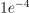.

verbosebool

Used for the online monitoring of the current basis updates (removed or added coefficients).

Notes

The cleaning strategy aims at building a PC expansion containing at most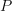significant coefficients, i.e. at mostsignificant basis functions. It proceeds as follows:

• Generate an initial PC basis made of thefirst polynomials (according to the adopted EnumerateFunction), or equivalently an initial set of indices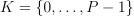.

• Discard from the basis all those polynomials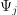associated with insignificance coefficients, i.e. the coefficients that satisfy: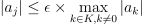whereis the significance factor, default is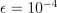.

• Add the next basis term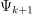to the current basis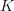.

• Reiterate the procedure until eitherterms have been retained or if the given maximum index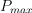has been reached.

Examples

>>> import openturns as ot
>>> ot.RandomGenerator.SetSeed(0)
>>> # Define the model
>>> inputDim = 1
>>> model = ot.SymbolicFunction(['x'], ['x*sin(x)'])
>>> # Create the input distribution
>>> distribution = ot.ComposedDistribution([ot.Uniform()]*inputDim)
>>> # Construction of the multivariate orthonormal basis
>>> polyColl = [0.0]*inputDim
>>> for i in range(distribution.getDimension()):
...     polyColl[i] = ot.StandardDistributionPolynomialFactory(distribution.getMarginal(i))
>>> enumerateFunction = ot.LinearEnumerateFunction(inputDim)
>>> productBasis = ot.OrthogonalProductPolynomialFactory(polyColl, enumerateFunction)
>>> # Truncature strategy of the multivariate orthonormal basis
>>> # We want to select, among the maximumDimension = 100 first polynomials of
>>> # the multivariate basis, those which have the maximumSize = 20 most
>>> # significant contribution (greatest coefficients), with respect to the
>>> # significance factor = 10^-4.
>>> maximumDimension = 100
>>> maximumSize = 20
>>> significanceFactor = 1e-4
...                                          maximumSize, significanceFactor)


Methods

 Compute initial basis for the approximation. Accessor to the underlying orthogonal basis. Accessor to the object's name. Accessor to the current vector index. Accessor to the object's id. Accessor to the maximum dimension of the orthogonal basis. Accessor to the maximum size of the orthogonal basis. Accessor to the object's name. Accessor to the orthogonal polynomials of the basis. Accessor to the object's shadowed id. Accessor to the significance factor. Accessor to the verbose. Accessor to the object's visibility state. Test if the object is named. Test if the object has a distinguishable name. setMaximumDimension(maximumDimension) Accessor to the maximum dimension of the orthogonal basis. setMaximumSize(maximumSize) Accessor to the maximum size of the orthogonal basis. setName(name) Accessor to the object's name. Accessor to the object's shadowed id. setSignificanceFactor(significanceFactor) Accessor to the significance factor. setVerbose(verbose) Accessor to the verbose. setVisibility(visible) Accessor to the object's visibility state. updateBasis(alpha_k, residual, relativeError) Update the basis for the next iteration of approximation.
__init__(*args)
computeInitialBasis()

Compute initial basis for the approximation.

getBasis()

Accessor to the underlying orthogonal basis.

Returns:
basisOrthogonalBasis

Orthogonal basis of which the adaptive strategy is based.

getClassName()

Accessor to the object’s name.

Returns:
class_namestr

The object class name (object.__class__.__name__).

getCurrentVectorIndex()

Accessor to the current vector index.

Returns:
indexinteger

Current index of the basis term.

getId()

Accessor to the object’s id.

Returns:
idint

Internal unique identifier.

getMaximumDimension()

Accessor to the maximum dimension of the orthogonal basis.

Returns:
Pinteger

Maximum dimension of the truncated basis.

getMaximumSize()

Accessor to the maximum size of the orthogonal basis.

Returns:
sizeinteger

Maximum number of significant terms of the basis.

getName()

Accessor to the object’s name.

Returns:
namestr

The name of the object.

getPsi()

Accessor to the orthogonal polynomials of the basis.

Returns:
polynomialslist of polynomials

Sequence ofanalytical polynomials.

Notes

The method computeInitialBasis() must be applied first.

Examples

>>> import openturns as ot
>>> productBasis = ot.OrthogonalProductPolynomialFactory([ot.HermiteFactory()])
[1,x0,-0.707107 + 0.707107 * x0^2]


Accessor to the object’s shadowed id.

Returns:
idint

Internal unique identifier.

getSignificanceFactor()

Accessor to the significance factor.

Returns:
factorfloat

Value of the significance factor.

getVerbose()

Accessor to the verbose.

Returns:
verbosebool

Return if the online monitoring of the current basis updates is enabled or not.

getVisibility()

Accessor to the object’s visibility state.

Returns:
visiblebool

Visibility flag.

hasName()

Test if the object is named.

Returns:
hasNamebool

True if the name is not empty.

hasVisibleName()

Test if the object has a distinguishable name.

Returns:
hasVisibleNamebool

True if the name is not empty and not the default one.

setMaximumDimension(maximumDimension)

Accessor to the maximum dimension of the orthogonal basis.

Parameters:
Pinteger

Maximum dimension of the truncated basis.

setMaximumSize(maximumSize)

Accessor to the maximum size of the orthogonal basis.

Parameters:
sizeinteger

Maximum number of significant terms of the basis.

setName(name)

Accessor to the object’s name.

Parameters:
namestr

The name of the object.

Accessor to the object’s shadowed id.

Parameters:
idint

Internal unique identifier.

setSignificanceFactor(significanceFactor)

Accessor to the significance factor.

Parameters:
factorfloat

Value of the significance factor.

setVerbose(verbose)

Accessor to the verbose.

Parameters:
verbosebool

Enable the online monitoring of the current basis updates or not.

setVisibility(visible)

Accessor to the object’s visibility state.

Parameters:
visiblebool

Visibility flag.

updateBasis(alpha_k, residual, relativeError)

Update the basis for the next iteration of approximation.

Notes

No changes are made to the basis in the fixed strategy.

## Examples using the class¶